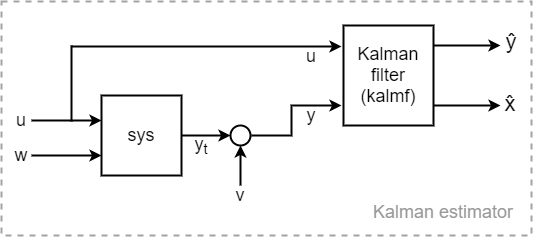# kalman

Kalman filter design, Kalman estimator

## Syntax

```[kest,L,P] = kalman(sys,Qn,Rn,Nn) [kest,L,P] = kalman(sys,Qn,Rn,Nn,sensors,known) [kest,L,P,M,Z] = kalman(sys,Qn,Rn,...,type) ```

## Description

`kalman ` designs a Kalman filter or Kalman state estimator given a state-space model of the plant and the process and measurement noise covariance data. The Kalman estimator provides the optimal solution to the following continuous or discrete estimation problems.

Continuous-Time Estimation

Given the continuous plant

`$\begin{array}{l}\stackrel{˙}{x}=Ax+Bu+Gw\text{ }\text{ }\text{ }\text{ }\left(\text{state}\text{\hspace{0.17em}}\text{equation}\right)\\ y=Cx+Du+Hw+v\text{ }\text{ }\text{ }\left(\text{measurement}\text{\hspace{0.17em}}\text{equation}\right)\end{array}$`

with known inputs u, white process noise w, and white measurement noise v satisfying

`$E\left(w\right)=E\left(v\right)=0,\text{ }E\left(w{w}^{T}\right)=Q,\text{ }E\left(v{v}^{T}\right)=R,\text{ }E\left(w{v}^{T}\right)=N$`

construct a state estimate $\stackrel{^}{x}\left(t\right)$ that minimizes the steady-state error covariance
$E\left(\left\{x-\stackrel{^}{x}\right\}{\left\{x-\stackrel{^}{x}\right\}}^{T}\right)$

The optimal solution is the Kalman filter with equations

`$\begin{array}{l}\stackrel{˙}{\stackrel{^}{x}}=A\stackrel{^}{x}+Bu+L\left(y-C\stackrel{^}{x}-Du\right)\\ \left[\begin{array}{c}\stackrel{^}{y}\\ \stackrel{^}{x}\end{array}\right]=\left[\begin{array}{c}C\\ I\end{array}\right]\stackrel{^}{x}+\left[\begin{array}{c}D\\ 0\end{array}\right]u\end{array}$`

The filter gain L is determined by solving an algebraic Riccati equation to be

`$L=\left(P{C}^{T}+\overline{N}\right){\overline{R}}^{-1}$`

where

`$\begin{array}{l}\overline{R}=R+HN+{N}^{T}{H}^{T}+HQ{H}^{T}\\ \overline{N}=G\left(Q{H}^{T}+N\right)\end{array}$`

and P solves the corresponding algebraic Riccati equation.

The estimator uses the known inputs u and the measurements y to generate the output and state estimates $\stackrel{^}{y}$ and $\stackrel{^}{x}$. Note that $\stackrel{^}{y}$ estimates the true plant output

`$y=Cx+Du+Hw+v$`Discrete-Time Estimation

Given the discrete plant

`$\begin{array}{l}x\left[n+1\right]=Ax\left[n\right]+Bu\left[n\right]+Gw\left[n\right]\\ y\left[n\right]=Cx\left[n\right]+Du\left[n\right]+Hw\left[n\right]+v\left[n\right]\end{array}$`

and the noise covariance data

`$E\left(w\left[n\right]w{\left[n\right]}^{T}\right)=Q,\text{ }E\left(v\left[n\right]v{\left[n\right]}^{T}\right)=R,\text{ }E\left(w\left[n\right]v{\left[n\right]}^{T}\right)=N$`

The estimator has the following state equation:

`$\stackrel{^}{x}\left[n+1|n\right]=A\stackrel{^}{x}\left[n|n-1\right]+Bu\left[n\right]+L\left(y\left[n\right]-C\stackrel{^}{x}\left[n|n-1\right]-Du\left[n\right]\right)$`

The gain matrix L is derived by solving a discrete Riccati equation to be

`$L=\left(AP{C}^{T}+\overline{N}\right){\left(CP{C}^{T}+\overline{R}\right)}^{-1}$`

where

`$\begin{array}{l}\overline{R}=R+HN+{N}^{T}{H}^{T}+HQ{H}^{T}\\ \overline{N}=G\left(Q{H}^{T}+N\right)\end{array}$`

There are two variants of discrete-time Kalman estimators:

• The current estimator generates output estimates $\stackrel{^}{y}\left[n|n\right]$ and state estimates $\stackrel{^}{x}\left[n|n\right]$ using all available measurements up to $y\left[n\right]$. This estimator has the output equation

`$\left[\begin{array}{c}\stackrel{^}{y}\left[n|n\right]\\ \stackrel{^}{x}\left[n|n\right]\end{array}\right]=\left[\begin{array}{c}\left(I-{M}_{y}\right)C\\ I-{M}_{x}C\end{array}\right]\stackrel{^}{x}\left[n|n-1\right]+\left[\begin{array}{cc}\left(I-{M}_{y}\right)D& {M}_{y}\\ -{M}_{x}D& {M}_{x}\end{array}\right]\left[\begin{array}{c}u\left[n\right]\\ y\left[n\right]\end{array}\right],$`

where the innovation gains Mx and My are defined as:

`$\begin{array}{c}{M}_{x}=P{C}^{T}{\left(CP{C}^{T}+\overline{R}\right)}^{-1},\\ {M}_{y}=\left(CP{C}^{T}+HQ{H}^{T}+HN\right){\left(CP{C}^{T}+\overline{R}\right)}^{-1}.\end{array}$`

Mx updates the prediction $\stackrel{^}{x}\left[n|n-1\right]$ using the new measurement $y\left[n\right]$.

`$\stackrel{^}{x}\left[n|n\right]=\stackrel{^}{x}\left[n|n-1\right]+{M}_{x}\underset{innovation}{\underbrace{\left(y\left[n\right]-C\stackrel{^}{x}\left[n|n-1\right]-Du\left[n\right]\right)}}.$`

When H = 0, ${M}_{y}=C{M}_{x}$ and $y\left[n|n\right]=Cx\left[n|n\right]+Du\left[n\right]$.

• The delayed estimator generates output estimates $\stackrel{^}{y}\left[n|n-1\right]$ and state estimates $\stackrel{^}{x}\left[n|n-1\right]$ using measurements only up to yv[n–1]. This estimator is easier to implement inside control loops and has the output equation

`$\left[\begin{array}{c}\stackrel{^}{y}\left[n|n-1\right]\\ \stackrel{^}{x}\left[n|n-1\right]\end{array}\right]=\left[\begin{array}{c}C\\ I\end{array}\right]\stackrel{^}{x}\left[n|n-1\right]+\left[\begin{array}{cc}D& 0\\ 0& 0\end{array}\right]\left[\begin{array}{c}u\left[n\right]\\ y\left[n\right]\end{array}\right]$`

`[kest,L,P] = kalman(sys,Qn,Rn,Nn)` creates a state-space model `kest` of the Kalman estimator given the plant model `sys` and the noise covariance data `Qn`, `Rn`, `Nn` (matrices Q, R, N described in Description). `sys` must be a state-space model with matrices $A,\left[B\text{\hspace{0.17em}}G\right],C,\left[D\text{\hspace{0.17em}}H\right]$.

The resulting estimator `kest` has inputs $\left[u;y\right]$ and outputs $\left[\stackrel{^}{y};\stackrel{^}{x}\right]$ (or their discrete-time counterparts). You can omit the last input argument `Nn` when N = 0.

The function `kalman` handles both continuous and discrete problems and produces a continuous estimator when `sys` is continuous and a discrete estimator otherwise. In continuous time, `kalman` also returns the Kalman gain `L` and the steady-state error covariance matrix `P`. `P` solves the associated Riccati equation.

`[kest,L,P] = kalman(sys,Qn,Rn,Nn,sensors,known)` handles the more general situation when

• Not all outputs of `sys` are measured.

• The disturbance inputs w are not the last inputs of `sys`.

The index vectors `sensors` and `known` specify which outputs y of `sys` are measured and which inputs u are known (deterministic). All other inputs of `sys` are assumed stochastic.

`[kest,L,P,M,Z] = kalman(sys,Qn,Rn,...,type)` specifies the estimator type for discrete-time plants `sys`. The `type` argument is either `'current'` (default) or `'delayed'`. For discrete-time plants, `kalman` returns the estimator and innovation gains L and M and the steady-state error covariances

`$\begin{array}{l}P=\underset{n\to \infty }{\mathrm{lim}}E\left(e\left[n|n-1\right]e{\left[}^{n}\right),\text{ }e\left[n|n-1\right]=x\left[n\right]-x\left[n|n-1\right]\\ Z=\underset{n\to \infty }{\mathrm{lim}}E\left(e\left[n|n\right]e{\left[}^{n}\right),\text{ }\text{ }\text{ }\text{\hspace{0.17em}}\text{\hspace{0.17em}}e\left[n|n\right]=x\left[n\right]-x\left[n|n\right]\end{array}$`

## Examples

See LQG Design for the x-Axis and Kalman Filtering for examples that use the `kalman` function.

## Limitations

The plant and noise data must satisfy:

• (C,A) detectable

• $\overline{R}>0$ and $\overline{Q}-\overline{N}{\overline{R}}^{-1}{\overline{N}}^{T}\ge 0$

• $\left(A-\overline{N}{\overline{R}}^{-1}C,\overline{Q}-\overline{N}{\overline{R}}^{-1}{\overline{N}}^{T}\right)$ has no uncontrollable mode on the imaginary axis (or unit circle in discrete time) with the notation

`$\begin{array}{l}\overline{Q}=GQ{G}^{T}\\ \overline{R}=R+HN+{N}^{T}{H}^{T}+HQ{H}^{T}\\ \overline{N}=G\left(Q{H}^{T}+N\right)\end{array}$`

## References

 Franklin, G.F., J.D. Powell, and M.L. Workman, Digital Control of Dynamic Systems, Second Edition, Addison-Wesley, 1990.

 Lewis, F., Optimal Estimation, John Wiley & Sons, Inc, 1986.

 Deshpande, A.S., "Bridging a Gap in Applied Kalman Filtering: Estimating Outputs When Measurements Are Correlated with the Process Noise." IEEE Control Systems Magazine, Vol. 37, Number 3, 2017, pp. 87–93.